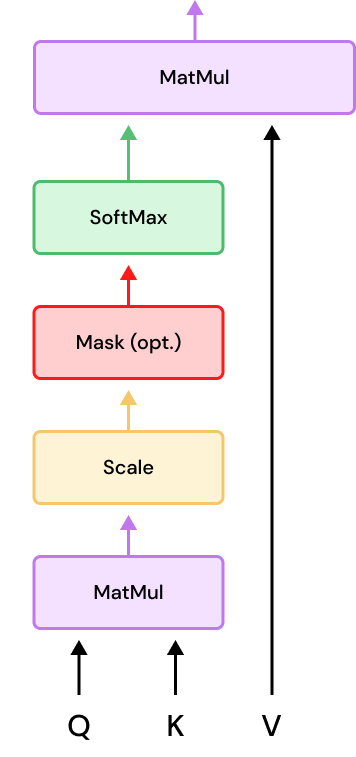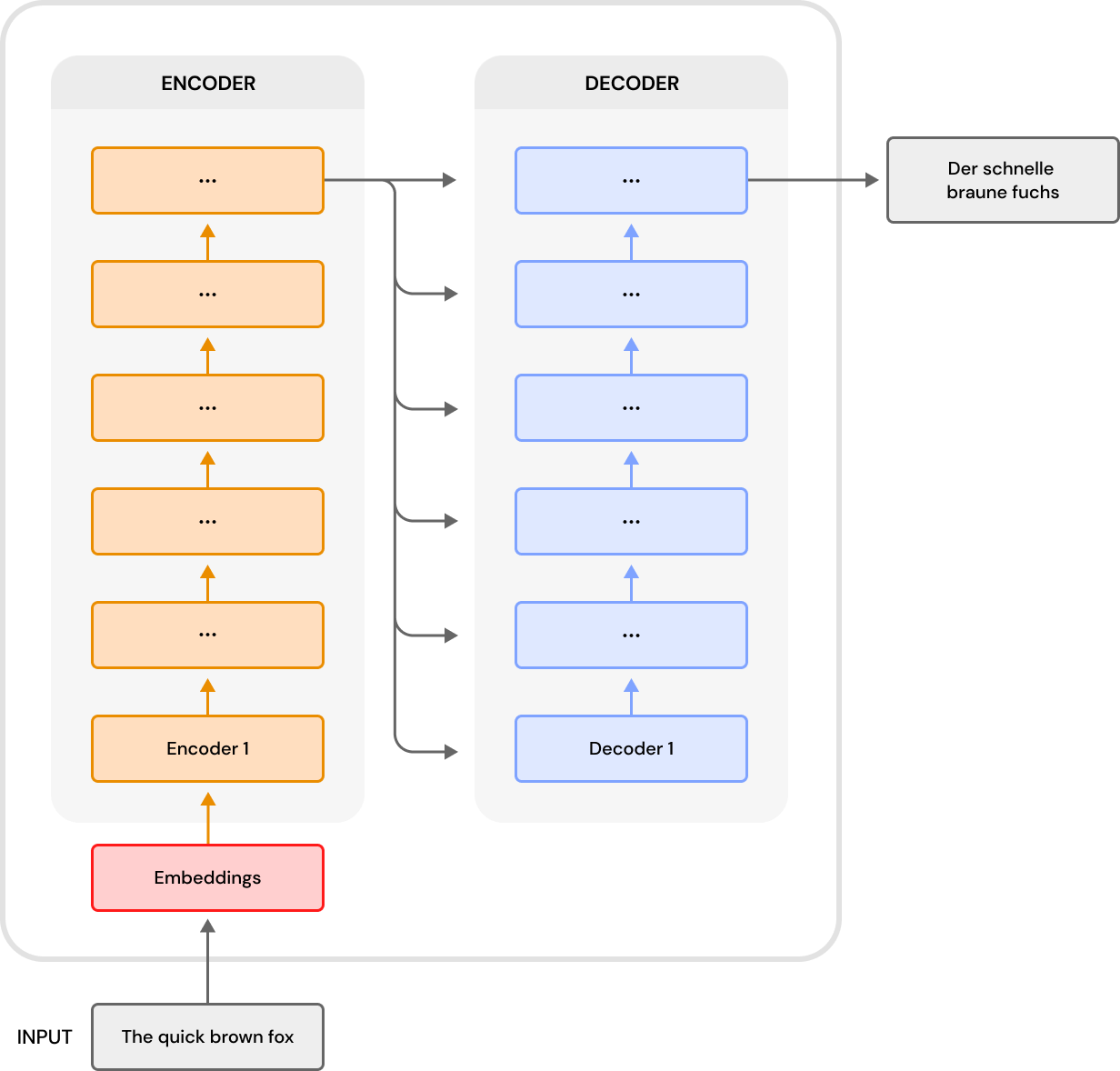# Transformers: The Nuts and Bolts

##### Technical Consultant

Gears, servos, and circuits, oh my! So, many things go into a Transformer! So many things to examine! Oh...wait...that’s the wrong Transformer.

Last time, we looked at what made the Transformer special (link). However, we did not look at its architecture. Let’s remedy that. It is going to be a lengthy post, so let’s get started.

### Overall Architecture

Vaswani et al. proposed the Transformer for machine translation. The Transformer’s overall architecture is an encoder-decoder one. Therefore, it consists of an encoder that feeds into a decoder. Both the encoder and decoder are composed of multiple layers/components.

### Encoder Stack

The encoder stack takes in the model inputs. Then, it maps them to abstract, continuous representations that hold the learned information for the inputs. The encoder consists of six encoders stacked on top of each other. Those layers sit on top of an embedding layer. Let’s see how the Transformer works by following a hypothetical input as it works its way through the Transformer, starting with a single encoder layer.

##### Embeddings and Positional Encoding

Let’s use a simple example to illustrate the workings of the Transformer. We’ll use “The quick brown fox” as our example input. So, the first thing we do is feed our input sentence into the model. The Transformer needs the embedding vectors for that input. That is what the embeddings module gets.

The embeddings module is a giant lookup table that holds the numerical embedding vectors for every word in the Transformer’s vocabulary. We take the embeddings from some other place (e.g., GloVe). So, the module looks up the embedding for each word and outputs that embedding.

The Transformer’s overall architecture is an encoder-decoder one.

But wait, there’s more. Sequential ordering matters in language and needs to be preserved. The sequential nature of RNNs preserves the sequential ordering of the input. The Transformer, however, isn’t naturally sequential. Therefore, we use positional encoding to retain the knowledge of the token positions. We add the positional encodings to the token embeddings. The equations to calculate the positional embeddings are:

PE₍ₚₒₛ,₂ₜ₎ = sin(pos/10000²ᵗ/ᵈₘ)

PE₍ₚₒₛ,₂ₜ₊₁₎ = cos(pos/10000²ᵗ/ᵈₘ)

So, for even position tokens, we use sine. Conversely, odd position tokens use cosine. dₘ is the dimension of the embeddings and positional embeddings. Next up is the multi-head self-attention mechanism.

##### Multi-Head Self-Attention Mechanism

The speed of the Transformer mainly comes from the multi-head self-attention layer. When we say that the Transformer is parallelizable, this is where that happens. We talked about the Transformer’s self-attention mechanism in the last post. In this post, we’ll focus on how to turn self-attention into multi-head self-attention.

The overall model uses dₘ-dimensional vectors. The vectors are broken up into smaller depth-dimensional vectors to enable processing them in parallel.

depth = dₘ / h

h is the number of attention heads. An attention head is just one processing unit of multi-head attention.

Then, we pass each set of depth-dimensional vectors to one of the attention heads for processing. The actual mechanism of this component is pretty straightforward. After splitting up the component’s inputs, we run each vector set (i.e., the depth-dimensional vectors) through 3 linear layers. Each layer has a different set of weights (wₐ, wₖ, wᵥ). The model initializes and trains the weights independently. The linear layers output the V, K, and Q matrices for their attention head. So, if the broken-up input embedding vectors are eᵢ, then the equations for these layers are:

Q = wₐeᵢ

K = wₖeᵢ

V = wᵥeᵢ

With the V, K, and Q matrices in hand, we apply the Transformer’s secret sauce to them (i.e., scaled dot-product attention).The self-attention layer is composed of many layers. The mask layer is present in the decoders, but not the encoders.

So, the self-attention layer is where we add parallelism to the Transformer. However, you have to get off the 6-lane highway at some point. That is where the Concat layer comes in. It brings the various self-attention lanes together. As the name suggests, the Concat layer concatenates the many outputs from the different self-attention layers. So instead of multiple matrices of depth-dimensional vectors, we get a single matrix of dₘ-dimensional vectors. The formula for the Concat layer is:

Afterward, a linear layer weights that single matrix with wₒ, producing the output of this layer. If we assume that the input to the linear layer is x, then the formula for that layer is:

Z = wₒx

There is one final step before the multi-head attention layer passes its outputs to the next layer. That step is we run the output of the multi-head attention layer through a residual and normalization layer. The purpose of this layer is to facilitate model training and stability. Starting with the residuals, a residual is just the difference between the observed and predicted values. Take, for example, the function f(xᵢ). It produces an output, xₒ = f(xᵢ). The residual is then just f(xᵢ) - xᵢ, or put more simply xₒ - xᵢ. In practice, that means we add the input to the layer to its output. In other words, if the layer’s input is Xₑ, then:

Zᵣ = Z + Xₑ

The residual and normalization layer facilitates training and model stabilization.

The residual provides a shortcut around the multi-head self-attention layer. It allows a path for gradients to flow without going through weights. That means that exploding and vanishing gradients are non-issues. After adding the residual, the model applies a layer normalization. The purpose of layer normalization is to stabilize the model during training. Models like the Transformer have multiple layers that we train via gradient descent. In other words, we compute an error for each training epoch. The layers update their weights according to that error. The layers do this updating one at a time. That presents a problem because once a layer has updated its weights, the error may no longer be accurate given the updated weights (i.e., covariate shift). As a result, the training that subsequent layers undergo may not be correct. So, model training for deeply layered networks is always chasing a moving target. Layer normalization solves this by normalizing the input to neural layers. That fixes the covariate shift problem. In this case, we normalize the output of the multi-head self-attention layer before moving it onto the next layer.

##### Position-wise Feed-Forward Layer

After the multi-head attention layer, we come to the position-wise feed-forward layer. This layer is two linear transformations with a ReLU activation in between. So, it is, essentially, two convolutions of kernel size 1. The position-wise feed-forward layer runs parallel instances of itself. Those processors operate on each position of the input matrix (i.e., word), hence “position-wise”. Each parallelized processor shares the weights, but each linear transform has its own set of weights. Its formula is:

FFN(x) = max(0, xW₁ + b₁)W₂ + b₂

The position-wise feed-forward layer has higher inner dimensionalities than input and output dimensionality.  That makes it reminiscent of the sparse-autoencoder. Overall, it is just a lot more matrix calculations. Following the position-wise feed-forward layer, we have another residual and normalization layer. Like the residual and normalization layer that follows the multi-head attention layer, this residual and normalization layer also facilitates training and model stabilization. This time, however, the outputs are stabilized for the subsequent encoder.6 stacked encoders make up the encoder stack. The same is true for decoders and the decoder stack.

So, that concludes our examination of a single encoder. As previously mentioned, 6 stacked encoders make up the encoder stack. The encoders feed into one another sequentially. The last encoder sends its outputs to the decoder stack.

### Decoder Stack

Even though this post is already very long, the decoders are almost identical to the encoders. So, we are in the home stretch. A decoder is composed of two multi-head attention layers and a position-wise feed-forward layer. Each of those sublayers also has its residual and normalization layer. Mostly, the layers are identical to their encoder counterparts. However, a different mission necessitates slight design changes. The decoder is autoregressive. In other words, it predicts a token sequence one token at a time. It considers the previously foretold tokens, the output from the encoder stack, and the current token for each prediction.

##### Shifted Inputs

The decoder shifts its inputs one position to the right by prepending a <start> token to the input sequence. Everything else works the same.

Sentences are sequential structures. Therefore, at time t, you have seen the word at time t and every word proceeding it. So when predicting a token sequence one token at a time, we can only look to past predictions. However, the unmodified multi-head attention allows you to look at the tokens after t. In other words, you can look into the future. Doing so for token prediction is ludicrous. It would be like someone who says they can predict next Tuesday’s lottery numbers. However, to do so, they consider the events of next Wednesday and Thursday.

Therefore, we need to change the first multi-head attention layer into a masked multi-head attention layer. We do this with a look ahead mask. A look ahead mask is a matrix of the same size as the matrix outputted by the multi-head attention layer. It contains 1s and negative infinities. The mask is applied to the scores after scaling but before the softmax. The softmax then just zeros out the future words for every word in the scaled matrix. The formula for this is simple enough.

The decoder is autoregressive.

Attention(Q, K, V) = softmax[[mask + (QKᵀ)] / √dₖ]V

##### Multi-head Attention With Memory

The second multi-head attention layer of the decoder is also slightly different from its encoder counterpart. The layer ingests an additional input, the Z output from the last encoder of the encoder stack. That Z output is memory. It comes in unmasked. The decoder incorporates it into the calculations of the K and V vectors. So this layer’s formulas are:

Q = wₐeᵢ

K = Zwₖeᵢ

V = Zwᵥeᵢ

Of course, the weights here are separate from the weights of the encoder’s multi-head attention.

### Let’s Cap it Off

So we have our encoder and decoder stacks. Now, all we need to do is add additional task-specific linear layers and a softmax. So if we are using the Transformer to translate into German, we add the linear layers for that task. The softmax is there to compute the probabilities over the outputs.

This post has been much longer than my typical ones. However, I think the extra length was worth it to understand the Transformer. Even though I typically post every Thursday, I will need to take a breather and skip next week.

Banner image credit to
vectorpocket
Natural Language Processing
Neural Networks